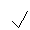gcsescience.com                                       29                                       gcsescience.com

Energy Transfer

Gravitational Potential Energy to Kinetic Energy.

When an object with Gravitational Potential Energy starts to fall,
its GPE is transferred into Kinetic Energy. The further
the object falls, the less GPE it has and the more KE it has.
When the object hits the ground,
all of its GPE has been transferred into KE.

Some processes transfer GPE to KE to GPE to KE and so on.
Examples are a bouncing ball, a pendulum and a comet.

If we know the Height of a Falling Object,
what will its Velocity be when it Hits the Ground?

If we look at the example of the 75 kg rock
4·0 metres
above the ground,
we can calculate that it has 3000J of GPE.

Using the equation for KE, we can
calculate how fast it is travelling when it hits the ground.
All of the rock's GPE has become KE,
so the rock has 3000J of KE when it hits the ground.

KE  =  ½mv2

3000 = 0·5 x 75 x v2

v2 = 3000 ÷ (0·5 x 75)
= 80

v80
= 8·94 m/s.

How much Time will a Falling Object take to Hit the Ground?

Since we now know how fast the rock was travelling,
we can calculate how long it took to fall the
4·0 metres.
Try it using the equation for acceleration.
a =
10 m/s2 (for gravity), v = 8·94 m/s, u = 0